# 6.5. XILab scripts¶

XILab scripting language is implemented using QtScript, which in turn is based on ECMAScript.

ECMAScript is the scripting language standardized by Ecma International in the ECMA-262 specification and ISO/IEC 16262.

QtScript (and, by extension, XILab) uses third edition of the ECMAScript standard .

## 6.5.1. Brief description of the language¶

### 6.5.1.1. Data Types¶

ECMAScript supports nine primitive data types. Values of type Reference, List, and Completion are used only as intermediate results of expression evaluation and cannot be stored as properties of objects. The rest of the types are:

• Undefined,
• Null,
• Boolean,
• String,
• Number,
• Object.

### 6.5.1.2. Statements¶

Most common ECMAScript language statements are summarized below:

Name Usage Description
Block {[<statement list>]} Several statements may be grouped into a block using braces.
Variable declaration var <varialble declaration list> Variables are declared using “var” keyword.
Empty statement ; Semicolon denotes an empty instruction. It is not required to end a line with a semicolon.
Conditional execution if (<condition>) <instruction> [ else <instruction> ] Conditional execution is done using “if … else” keywords. If a condition is true, then “if”-block instruction is executed, else an “else”-block instruction is executed.
Loop do <loop body> while (<condition>) while (<condition>) <loop body> for ([<initialization>]; [<condition>]; [<iterative statement>]) <loop body> Loops have several forms. A “do … while …” loop executes loop body and then checks if condition is true or false to see whether it should stop or continue running. A “while … do …” loop repeatedly checks the condition and executes loop body if it is true. A “for …” loop executes an initialization statement once, then executes an iterative statement and loop body while the condition is true.
Return return [<expression>] Stops function execution and returns expression as a result.
Exception throw <expression> Generates or “throws” an exception, which may be processed by the “try” statement (see below).
Try-catch block try <block> catch (<identifier>) <block> try <block> finally <block> try <block> catch (<identifier>) <block> finally <block> Used together with exceptions. This statement tries to execute its “try”-block. If an exception is thrown in it, then a “catch”-block is executed. Finally a “finally”-block is executed unconditionally. Either a “catch” or a “finally” block may be omitted.

### 6.5.1.3. Variable statements¶

Variables are declared using var keyword. A declared variable is placed within visibility scope that corresponds to the function in which it is declared. If the variable is declared outside of functions, it is placed in the global visibility scope. Variable is created when the function within which it was declared, or, if the variable is global, at the start of the application. When a variable is created it is initialized with Undefined value. If a variable is created with initialization, the initialization does not occur in the moment of variable creation, it happens when the string with the var statement executes.

### 6.5.1.4. Reserved words¶

The following words are the reserved keywords in the language and may not be used as identifiers:

break     else        new     var
case      finally     return  void
catch     for         switch  while
continue  function    this    with
default   if          throw
delete    in          try
do        instanceof  typeof


The following words are used as keywords in proposed extensions and are therefore reserved to allow for the possibility of future adoption of those extensions:

abstract  enum        int        short
boolean   export      interface  static
byte      extends     long       super
char      final       native     synchronized
class     float       package    throws
const     goto        private    transient
debugger  implements  protected  volatile
double    import      public


### 6.5.1.5. Functions¶

Functions are objects in ECMAScript. Functions like any other objects can be stored in variables, objects and arrays, can be passed as arguments to other functions and can be returned by functions. Functions, like any other objects may have properties. Essential specific feature of functions is that they can be invoked.

In the application text, the most common way to define a function is:

function sum(arg1, arg2) { // a function which takes two parameters
return arg1 + arg2;    // and returns their sum
}


## 6.5.2. Syntax highlighting¶

Script window text has syntax highlighting. Its colors are:

Statement type color text example
Arbitrary functions purpleXILab functions blue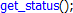Positive numbers green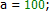Negative numbers red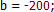Comments grey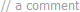The rest of the text black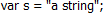During the script execution the background of line with the last executed command is changed to dark gray with update rate of once in every 20 ms.

This image shows XILab functions which are available from scripts, aside from standard built-in language functions.• log(string text [, int loglevel]) – save text to the XILab log
• msleep(int ms) - delay script execution
• new_axis(int serial_number) - create new axis object
• new_file(string filename) - create new file object
• new_calibration(int A, int Microstep) - create calibration structure to pass to calibrated functions
• get_next_serial(int serial) - get next serial out of an ordered list of opened controller serials
• command_wait_for_stop(int refresh_period) - wait until controller stops moving
• and all libximc library functions (see Programming guide)

Also, all constant values from the communication protocol are defined and can be used in scripts. Usage example.

### 6.5.3.1. XILab log¶

Logging is done by calling log(string text [, int loglevel] ) function. This function adds the text line to the XILab log. If the second loglevel parameter is passed the message receives the appropriate logging level and is displayed in corresponding color.

Loglevel Type
1 Error
2 Warning
3 Info

Example:

var x = 5;
log("x = " + x);


Function usage example

Note: It is not recommended to invoke functions that interact with XILab user interface (i.e. logging function) with a frequency of more than once in 20 ms.

### 6.5.3.2. Script execution delay¶

Script is paused by calling the msleep(int ms) function, which suspends script execution for ms milliseconds.

Example:

msleep(200);


Function usage example.

### 6.5.3.3. New axis object creation¶

XILab multi-axis interface provides the ability to manage controllers via scripts. The difference from the single-axis case is that you should specify the controller which receives the command. An “axis” object is introduced to abstract this concept. It has methods which match the libximc library function names. Controllers are identified by their serial numbers.

Example:

var x = new_axis(123);
x.command_move(50);


In this example first line of the script creates an axis-type object with the variable name “x”, which tries to use controller with the serial number “123”. If this controller is not connected, then the script will return an error and terminate. The second line of the script sends a “move to position 50” command to this controller.

Function usage example.

### 6.5.3.4. New file object creation¶

XILab scripts can read from and write to files. To do this you need to create a “file” object, passing desired filename in its constructor. File object has the following functions:

 return_type Function_name Description bool open() Opens the file. File is opened in read-write mode if possible, in read-only mode otherwise. void close() Closes the file. Number size() Returns file size in bytes. bool seek(Number pos) Sets current position in file to pos bytes . bool resize(Number size) Resizes the file to size bytes. If size is less than current file size, then the file is truncated, if it is greater than current file size, then the file is padded with zero bytes. bool remove() Removes the file. String read(Number maxsize) Reads up to maxsize bytes from the file and returns result as a string. Data is read in utf-8 Unicode encoding. Number write(String s, Number maxsize) Writes up to maxsize btyes to the file from the string. Data is written in utf-8 unicode encoding, end-of-line character should be set by user. Returns amount of written bytes or -1 if an error occurred.

All file functions which return bool type, return “true” on success and “false” on failure.

Use “/” symbol as path separator, this works on all systems (Windows/Linux/Mac).

Example:

var winf = new_file("C:/file.txt"); // An example of file name and path on Windows
var linf = new_file("/home/user/Desktop/file.txt"); // An example of file name and path on Linux
var macf = new_file("/Users/macuser/file.txt"); // An example of file name and path on Mac

var f = winf; // Pick a file name
if (f.open()) { // Try to open the file
f.write( "some text" ); // If successful, then write desired data to the file
f.close(); // Close the file
} else { // If file open failed for some reason
log( "Failed opening file" ); // Log an error
}


Function usage example.

### 6.5.3.5. Creation of calibration structure¶

new_calibration(double A, int Microstep) function takes as a parameter a floating point number A, which sets the ratio of user units to motor steps, and microstep division mode, which was either read earlier from MicrostepMode field of get_engine_settings() return type, or set by a MICROSTEP_MODE_ constant. This function returns calibration_t structure, which should be passed to calibrated get_/set* functions to get or set values in user units. The following two forms are functionally equivalent:

// create calibration: type 1
var calb = new_calibration(c1, c2);

// create calibration: type 2
var calb = new Object();
calb.A = c1;
calb.MicrostepMode = c2;


Function usage example.

### 6.5.3.6. Get next serial¶

get_next_serial(int serial) function takes as a parameter an integer number and returns the smallest serial from a sorted list of opened controller serials which is strictly greater than the parameter. If there are no such serials a zero is returned. This function is a convenient shortcut for automatic creation of “axis” type objects without hardcoded serial numbers.

Example:

var first_serial = get_next_serial(0);
var x = new_axis(first_serial);
var y = new_axis(get_next_serial(first_serial));


In this example in the first line we obtain a serial, in the second line an axis-type object is created, in the third line we get the next serial and create an axis for it.

Function usage example.

### 6.5.3.7. Wait for stop¶

The command_wait_for_stop(int refresh period) script function waits until the controller stops movement, that is, until the MVCMD_RUNNING bit in the MvCmdSts member of the structure returned by the get_status() function becomes unset. command_wait_for_stop script function uses command_wait_for_stop libximc function and takes as a paramater an integer denoting time delay in milliseconds between successive queries of controller state.

This function is also present as a method of an “axis”-type object.

Function usage example.

### 6.5.3.8. libximc library functions¶

Libximc library functions with “get*” prefix read settings from the controller and return the corresponding settings structure. Libximc library functions with “set*” prefix take as a parameter a settings data structure and write these settings to the controller. There are two ways to set data structure contents:

// set settings: type 1
var m = get_move_settings();
m.Speed = 100;
set_move_settings(m);


2. create an Object and set all of its properties that are present as members of the data structure (case-sensitive).

// set settings: type 2
var m = new Object;
m.Speed = 100;
m.uSpeed = 0;
m.Accel = 300;
m.Decel = 500;
m.AntiplaySpeed = 10;
m.uAntiplaySpeed = 0;
set_move_settings(m);


Please note, that in the first case controller receives an additional command (sent by the get-function before the set-). In the second case one should initialize all object properties corresponding to structure members. Any missing property will be initialized with zero. Any property that does not match a structure member name will be ignored. Any property with non-matching type will be typecast according to EcmaScript rules. All data structures are described in Communication protocol specification chapter of the manual.

Function usage example.

## 6.5.4. Examples¶

This section contains examples of typical tasks which can be easily automated by XILab scripts.

### 6.5.4.1. Cyclic movement script¶

var first_border = -10; // first border coordinate in mm
var second_border = 10; // second border coordinate in mm
var mm_per_step = 0.005; // steps to distance translation coefficient
var delay = 100; // delay in milliseconds
var calb = new_calibration(mm_per_step, get_engine_settings().MicrostepMode); // create calibration structure
command_stop(); // send STOP command (does immediate stop)
command_zero(); // send ZERO command (sets current position and encoder value to zero)
while (1) { // infinite loop
command_move_calb(first_border, calb); // move towards one border
command_wait_for_stop(delay); // wait until controller stops moving
command_move_calb(second_border, calb); // move towards another border
command_wait_for_stop(delay); // wait until controller stops moving
}


### 6.5.4.2. A script which scans and writes data to the file¶

var start = 0; // Starting coordinate in steps
var step = 10; // Shift amount in steps
var end = 100; // Ending coordinate in steps

var speed = 300; // maximum movement speed in steps / second
var accel = 100; // acceleration value in steps / second^2
var decel = 100; // deceleration value in steps / second^2
var delay = 100;

var m = get_move_settings(); // read movement settings from the controller
m.Speed = speed; // set movement speed
m.Accel = accel; // set acceleration
m.Decel = decel; // set deceleration
set_move_settings(m); // write movement settings into the controller

var f = new_file("C:/a.csv"); // Choose a file name and path
f.open(); // Open a file
f.seek( 0 ); // Seek to the beginning of the file

command_move(start); // Move to the starting position
command_wait_for_stop(delay); // Wait until controller stops moving

while (get_status().CurPosition < end) {
f.write( get_status().CurPosition + "," + get_chart_data().Pot + "," + Date.now() + "\n" ); // Get current position, potentiometer value and date and write them to file
command_movr(step); // Move to the next position
command_wait_for_stop(delay); // Wait until controller stops moving
}
f.close(); // Close the file


### 6.5.4.3. A script which moves the controller through the list of positions with pauses¶

var axis = new_axis(get_next_serial(0)); // Use first available controller
var x; // A helper variable, represents coordinate
var ms; // A helper variable, represents wait time in milliseconds
var f = new_file("./move_and_sleep.csv"); // Choose a file name and path; this script uses a file from examples in the installation directory
f.open(); // Open a file
while ( str = f.read(4096) ) { // Read file contents string by string, assuming each string is less than 4 KiB long
var ar = str.split(","); // Split the string into substrings with comma as a separator; the result is an array of strings
x = ar; // Variable assignment
ms = ar; // Variable assignment
log( "Moving to coordinate " + x ); // Log the event
axis.command_move(x); // Move to the position
axis.command_wait_for_stop(100); // Wait until the movement is complete
log( "Waiting for " + ms + " ms" ); // Log the event
msleep(ms); // Wait for the specified amount of time
}
log ( "The end." );
f.close(); // Close the file


move_and_sleep.csv

• a sample file for use with the above example

### 6.5.4.4. A script which enumerates all available axes and gets their coordinates¶

var i = 0; // Declare loop iteration variable
var serial = 0; // Declare serial number variable
var axes = Array(); // Declare axes array
while (true) { // The loop
serial = get_next_serial(serial); // Get next serial
if (serial == 0) // If there are no more controllers then...
break; // ...break out of the loop
var a = new Object(); // Create an object
a.serial = serial; // Assign serial number to its "serial" property
a.handle = new_axis(serial); // Assign new axis object to its "handle" property
axes[i] = a; // Add it to the array
i++; // Increment counter
}
for (var k=0; k < axes.length; k++) { // Iterate through array elements
log ( "Axis with S/N " + axes[k].serial + " is in position " + axes[k].handle.get_status().CurPosition ); // For each element print saved axis serial and call a get_status() function
}


/*
*/
var a = new_axis(get_next_serial(0)); // take first found axis
var gets = a.get_status(); // read status once and reuse it

var gpio = gets.GPIOFlags;
var left = STATE_LEFT_EDGE;
var right = STATE_RIGHT_EDGE;
var mask = left | right;
var result = gpio & mask;
log( to_binary(left) + " = left limit switch flag" );
log( to_binary(right) + " = right limit switch flag" );
log( to_binary(mask) + " = OR operation on flags gives the mask" );
log( to_binary(gpio) + " = gpio state" );
log( to_binary(result) + " = AND operation on state and mask gives result" );
if ( result ) {
log("At least one limit switch is on");
} else {
log("Both limit switches are off");
}

// Binary representation function
function to_binary(i)
{
bits = 32;
x = i >>> 0; // coerce to unsigned in case we need to print negative ints
str = x.toString(2); // the binary representation string
return (repeat("0", bits) + str).slice (-bits); // pad with zeroes and return
}

// String repeat function
function repeat(str, times)
{
var result="";
var pattern=str;
while (times > 0) {
if (times&1) {
result+=pattern;
}
times>>=1;
pattern+=pattern;
}
return result;
}

  Seeking beyond the end of a file: If the position is beyond the end of a file, then seek() shall not immediately extend the file. If a write is performed at this position, then the file shall be extended. The content of the file between the previous end of file and the newly written data is UNDEFINED and varies between platforms and file systems.